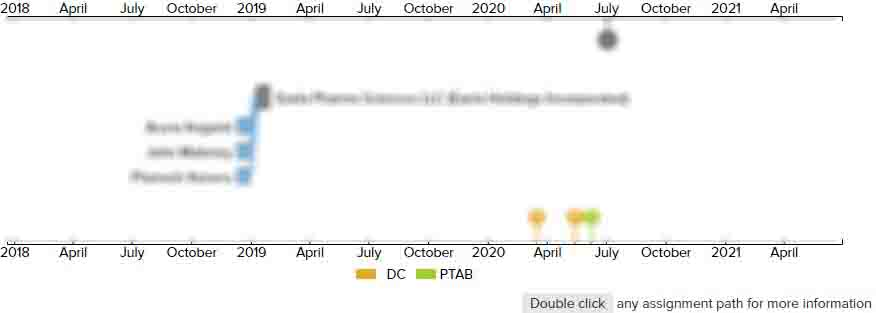# 6-DOF subject-monitoring device and method

DC
• US 6,997,882 B1
• Filed: 12/21/2002
• Issued: 02/14/2006
• Est. Priority Date: 12/21/2001
• Status: Expired due to Term
##### First Claim
Patent Images

1. A method of monitoring a subject during a monitoring period, said method comprising the steps of:

• (a) attaching at least one array of accelerometer modules to at least one body-segment of the subject, the accelerometer modules having an aggregate of at least six axes of measurement;

(b) positioning the subject in at least three substantially stationary calibration poses, the calibration poses being sufficiently different from one another to produce appreciable differences in acceleration signals produced by the array of step (a);

(c) acquiring acceleration signals from the array of step (a) for each of the calibration poses of step (b), said acceleration signals representing the accelerations of the body-segment relative to each of the x, y, and z-axes of an anatomical reference frame;

(d) averaging the acceleration signals acquired at step (c) to minimize the effects of noise and involuntary motion;

(e) acquiring a gravity vector value from the averaged acceleration signals obtained at step (d);

(f) applying the gravity vector value acquired at step (e) to the acceleration signals obtained at step (c) to obtain a correction factor for correcting the orientation of each axis of measurement relative to the anatomical reference-frame;

(g) acquiring from the array of step (a) acceleration signals representing the accelerations of the body-segment movements relative to each of the x, y, and z—

axes of an anatomical reference-frame;

(h) processing the acceleration signals acquired at step (g) to obtain 6-DOF data;

(i) processing the 6-DOF data obtained at step (h) by incorporating the correction factor obtained at step (f) to obtain information regarding the body-segment movement with respect to the x, y, and z-axes of an inertial reference-frame; and

(j)) displaying the body-segment movement information obtained at step (i).

• ##### 4 Assignments
Timeline View
Assignment View×
×